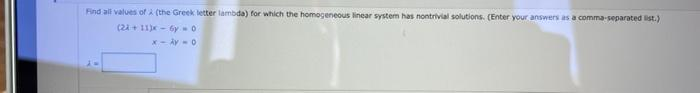Home / Expert Answers / Algebra / find-alf-values-of-lambda-the-greek-letter-lambda-for-which-the-homogeneous-linear-system-pa316

# (Solved): Find alf values of $$\lambda$$ (the Greek letter lambda) for which the homogeneous linear system ...Find alf values of $$\lambda$$ (the Greek letter lambda) for which the homogeneous linear system has nontrival solutions. (Enter your answers as a comma.separated ist.) $\begin{array}{r} (2 i+11) x-6 y=0 \\ x-\lambda y=0 \end{array}$

We have an Answer from Expert